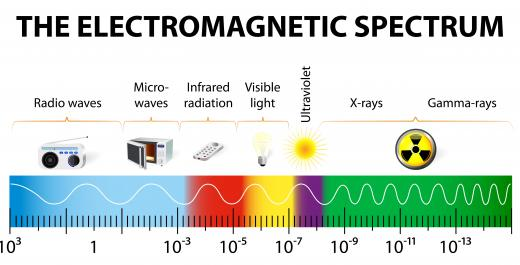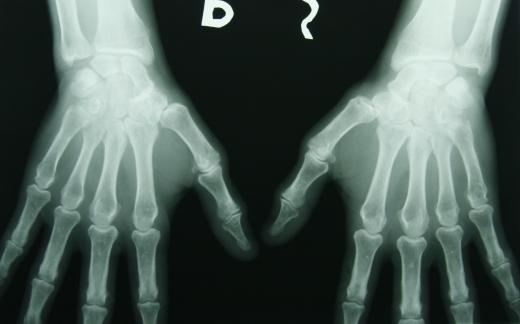# What Is Electromagnetic Frequency?

Alex Paul
Alex PaulThe electromagnetic spectrum is made up of the range of possible wave frequencies.

Electromagnetic frequency is a measure of how many times the peak of a wave passes a particular point each second. It is measured in Hertz, which also can be written as simply “per second”. The frequency of a wave is one of its most fundamental principles, and the range of possible frequencies makes up something known as the electromagnetic spectrum. This runs from low-energy radio waves to high-energy gamma rays. Unlike its wavelength, the electromagnetic frequency of a wave doesn’t change.

Electromagnetic radiation is a type of wave that travels at the speed of light. It is a transverse wave, which means it oscillates up and down in a direction opposite that in which it is moving. The electromagnetic frequency of a wave is defined as how many times the peak of this oscillation moves past a point each second. This has a major effect on the properties of the wave, including its energy. The wavelength, on the other hand, is the distance between two peaks of the wave or, in other words, the length of a full cycle.X-rays are high electromagnetic frequency waves used to look inside the human body.

A wave's electromagnetic frequency is directly related to the amount of energy carried by the wave. Low-frequency electromagnetic waves, for example, have small amounts of energy and, therefore, are relatively safe. These are more commonly known as radio waves. Low-frequency waves, such as radio waves and microwaves, have long wavelengths.

If the electromagnetic frequency of a wave is high, then the wave carries a large amount of energy. Conversely, the wavelength of the wave in this situation is very short. X-rays and gamma rays are two examples of high electromagnetic frequency waves, which is why these types are dangerous when humans are exposed to them. Visible light also is a type of electromagnetic wave with a frequency somewhere around the middle of the electromagnetic spectrum.

When a wave passes from one medium to another, such as from air to water, it changes direction as the result of a phenomenon known as refraction. This is because the wave changes speed as it enters a material with a different density. A common mistake is to assume that this changes the electromagnetic frequency of the wave. This is not the case, because the frequency of a wave stays the same, regardless of the medium. It is the wavelength and speed of the wave that change, resulting in a slower wave of the same energy.